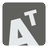Skew-symmetric Matrix
Elementary
English HU JA RU

In mathematics, particularly in linear algebra, a skew-symmetric matrix (also known as an antisymmetric or antimetric) is a square matrix A which is transposed and negative. This means that it satisfies the equation A = −AT. If the entry in the i-th row and j-th column is aij, i.e. A = (aij) then the symmetric condition becomes aij = −aji.

You should determine whether the specified square matrix is skew-symmetric or not.

You can find more details on...

You should be an authorized user in order to see the full description and start solving this mission.